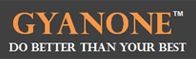# Interest Problems

 Categories : Interest ProblemsQ) After adding a simple interest of \$ 270 an investment becomes \$1395. If the principal was invested at 6% p.a. simple interest, for how long was the principal invested.A) 3 YearsB) 4 YearsC) 5 YearsD) 6 YearsE) 7 YearsSolution: As a Simple Interest problem, this question would make use of the formula,SI = Principal * Rate * TimeAmount = SI + PrincipalPlugging in values, we get,\$1395 = \$ 270 + PrincipalPrincipal = \$1395 - \$270 => \$1125Using the formula to calculate time period, we get,\$270 = \$1125*0.06*Time=> Time = 4 yearsCorrect Answer: BAbout the AuthorGyanOne is an educational services firm focused on premium GMAT coaching, application... Categories : Interest Problems, Problem SolvingSimple interest and compound interest - essential topics for an MBA. GMAC thinks the same too. So you will find these questions randomly distributed in your GMAT Exam. Simple interest is the most basic and is a function of P, the principle amount of money invested, the interest rate earned on the principle, i, and the amount of time the money is invested, t (this is usually stated in periods, such as years or months). The resulting equation is:Interest = iPtIn basic terms, the above equation tells us the amount of interest that would be earned on a principle amount invested (P), for a given time (t) at a given interest rate (i).ExampleIf you invested \$1,000 (P = your principle) for one year (t = one year) at 6% simple interest (i = given interest rate), you would get \$60 in interest at the end of the year and would have a total of \$1,060.For compound interest, you would earn slightly more. Let’s look at similar type problem, though this one involves compound interest.... Categories : Interest Problems One of the topics commonly tested in the GMAT Quant section is that of Simple Interest. While the formulas and concepts applied herein are simple enough, a lot of test takers get caught up in the terminology surrounding the concepts and therefore get their answers on this topic wrong. Here, then, are the concepts on Simple Interest that you can get tested on when you take the GMAT, including the terminology: Simple Interest = P x R x T/100 Where P: Principal (or the amount originally lent out) R: Rate of interest (expressed in terms of %) T: Time period for which the loan is madeCommon mistakes made by test takers when dealing with Simple Interest problems1. Taking the rate of interest in decimals, instead of in percentage terms: Remember, the 100 in the denominator of the formula is there because it assumes that the rate of interest (R) will be taken in percentage terms, not in decimals. Example: If P = \$1000, R = 4% per annum, and T = 3 years, SI = 1000 x 4 x 3 / 100 = 120 Do NOT take SI = 1000 x 0.04 x 3 / 100 = 1.2 2. Interpreting SI to...

Get F1GMAT's Newsletters (Best in the Industry)

• Ranking Analysis
• Post-MBA Salary Trends
• Post-MBA Job Function & Industry Analysis
• Post-MBA City Review
• MBA Application Essay Tips
• School Specific Essay Tips
• GMAT Preparation Tips